In this article we will return to our scientific and geometric investigation of the universe.

We will focus on the geometry of the Earth for three articles, then we will move into the geometry of the solar system for six articles.  After that we will finish with a three-part series on the geometry of galaxies and galactic clusters.

Before we begin we will briefly review the Platonic solids here.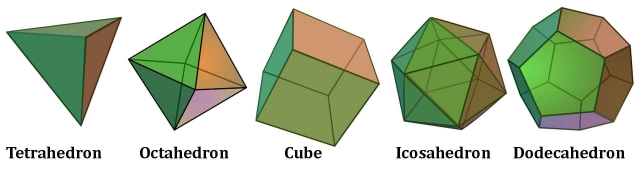Regular Polygons

Regular polygons are 2D shapes that have equal angles and equal side lengths.  These are listed in the chart below.

 Shape Sides Sum of Interior Angles Each Angle Triangle 3 180 60 Square 4 360 90 Pentagon 5 540 108 Hexagon 6 720 120 Heptagon 7 900 128.57… Octagon 8 1080 135 Nonagon 9 1260 140 Decagon 10 1440 144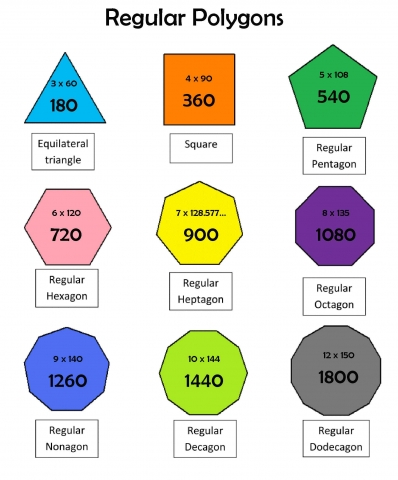Regular Polyhedra (The 5 Platonic Solids)

Regular polyhedrons (the five 3D Platonic solids) have equal regular polygon faces and identical vertices.

The Platonic solids are three-dimensional volumes, or ‘regular polyhedrons’ made up of three ‘regular polygons’ including the triangle, square and pentagon.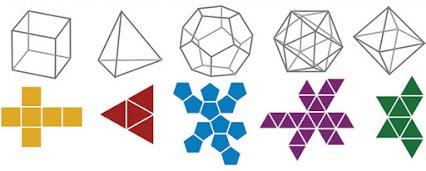These five solids are extremely important shapes.  Along with the circle/sphere and the regular polygons they are the most important geometric shapes to understand.

They are the only shapes:

• with equal side lengths
• with equal interior angles
• that look the same from each vertex (corner point)
• with faces made of the same regular polygon (triangle, square, pentagon) 3, 4, 5
• that all fit perfectly in a sphere (circumsphere) with all points resting on the circumference (This means the Platonic solids will all fit exactly in an appropriately sized sphere with all points exactly touching the circumference.)

 Plato’s Element Volume Shape of Face Faces Edges Corners Sum of Angles Dual “Heaven” or Aether Dodecahedron pentagon 12 30 20 6480 Icosahedron Fire Tetrahedron triangle 4 6 4 720 Tetrahedron Air Octahedron triangle 8 12 6 1440 Cube Water Icosahedron triangle 20 30 12 3600 Dodecahedron Earth Cube square 6 12 8 2160 Octahedron Star Tetrahedron Triangle 1440

In previous articles we have seen how the Platonic solids show up on the subquantum (Aether unit) scale, the quantum scale of subatomic particle interactions, the geometry of light and the photon, the geometry of sound and vibration, the geometry of the atom and the periodic table of elements, the geometry of molecules, water, DNA and ice, the geometry of minerals and crystals, the geometry of life forms including plants, fungi, viruses, bacteria, plankton, sea creatures, insects, animals, and the human body.

We will move on from there to show how the Platonic solids show up on the planetary, solar, galactic and universal levels.

In short, the Platonic solids are the underpinning geometric patterns, or seeds, of physical reality on all scales, without exception.  They clearly illustrate that there is a harmonic unity to all of physical reality.Due to the infinite variations of all physical life, these geometric patterns can sometimes be discerned easily and other times not so easily.  Yet no matter how veiled the patterns and proportions can be, they are always present, showing us the inherent order and unity that binds all things.

Within order there is freedom of expression.  This is a law of the universe.  Therefore, we can see infinite variations on the same basic geometric themes across all scales of life and matter.

Reviewing Article 4, these Platonic solid numbers (sum of the angles) show up in many ways.  One way is through the Measure of Time.

Time Measure

Earth Motion #1 – rotation about the axis                  (day cycle: 24 hours)

Earth Motion #2 – revolution around the sun             (year cycle: 365.242 days)

Earth Motion #3 – Precession of the Equinoxes         (galactic cycle: 25920 years)• One solar day =          24 hours
• 60 seconds x 60 min =      3600 seconds/hour
• 24 hours x 60 min =          1440 minutes/day
• 1440 min x 60 sec =          86400 seconds/day
• 720 min x 60 sec =            43200 seconds/half day (division of light and dark hours)

• 25920 seconds =      432 minutes
• 432 minutes =          2 hours
• 12960 seconds =      216 minutes
• 6480 seconds =       108 minutes
• 25920 hours =         1080 days
• 25920 days =           72 years (of 360 day sacred calendar)

• Current average human lifespan = 72 years (1 day of the Great Year)
• Average human resting heartbeat = 72 bpm
• “Room temperature” = 72° F

Precession of the Equinoxes (See Article 257)

• 1 Great Year =          25,920 years
• 1 Platonic Month =          2160 years
• Number of Zodiacal Ages in the Great Year = 12
• Number of Degrees allocated to each Age = 30º
• Number of years for a Precessional shift along 1 degree = 72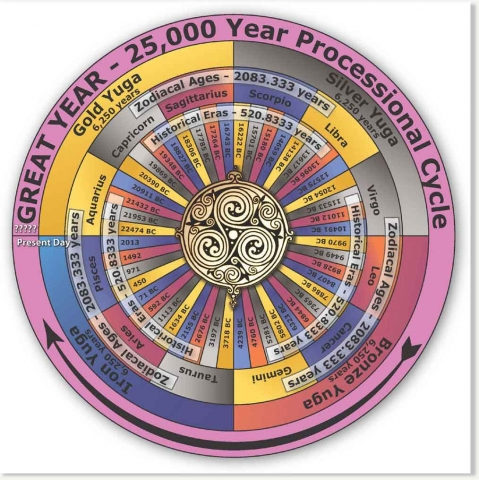Keep noting the harmony and order inherent in the system of Time and how Time itself is related to Geometry (the simple shapes of the regular polygons and polyhedra).  Time is simply motion through geometry!

Another way the Platonic solid numbers show up is through Space (or Distance) Measure.

Space Measure• One foot =                            12 inches
• One square foot =     144 sq inches
• One cubic foot =        1728 cubic inches  (864 x 2 = 1728; 72 x 12 = 864)
• One square yard =    1296 inches     (648 x 2 = 1296/ 72 x 9 = 648)
• One cubic yard =       27 cubic feet

Origin of the mile• from Latin mil = thousand = 1000 paces
• 1 pace = a step with right and left foot
• Each pace averages 5.28 feet
• 1 mile = 5280 feet (1000 paces of 5.28 feet)
• 5 miles = 7920 feet (72 x 110 = 7920)
• 7920 miles is the diameter of the Earth

Astronomical Units (AU) & Light Years (LY)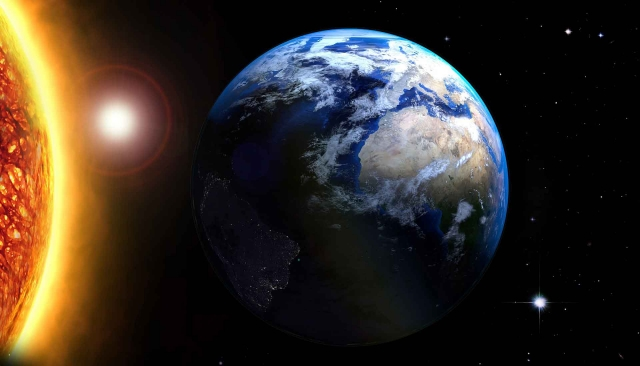An inch is to a mile as an astronomical unit is to a light-year.  (99.8% accuracy)

• 1 AU = the average distance the Earth is from the Sun
• 1 LY = the total distance light travels during 1 Earth revolution around the Sun
• 63,360 inches = 1 mile
• 63,241.1 AU = 1 LY

Origin of the meter

“Originally the meter was designed to be one ten-millionth (1/10,000,000) of a quadrant, the distance between the Equator and the North Pole.  Using this unit, the circumference of perfectly round Earth should be exactly 40,000,000 meters (40,000 km).  Today, official value of the Earth’s circumference along the line of longitude is 40,007.86 km.”1Here we would like to remind readers that in Cosmic Core we do not assume that one system of measurement is superior to another.  There exists a great amount of scorn and derision heaped up the standard system of measurement because it is still used in the United States over the metric system.  We believe that each system of measurement has its place in the body of knowledge called metrology.  There is no competition as to which is better.  They are both useful and interesting and not only do we advocate learning both systems, but we also advocate not belittling one against the other.

Earth Metrology

Metrology is the science of measurement.  Units of measure we use today are connected with the size and movements of our planet.

The same applies to the ancient units of measure.

Randall Carlson explains, “In Historical Metrology Algernon E. Berriman demonstrated the link between ancient systems of measurement and the Earth as a planet, its dimensions and geometry.  By doing so he provided a crucial link in the chain of interrelated processes unifying man, temple, planet and cosmos.”The nautical mile is based on the circumference of the Earth.

“If you divide the circumference of the Earth into 360 degrees and then divide each degree into 60 minutes you will get 21,600 minutes of arc.  1 nautical mile is defined as 1 minute of arc (of the circumference of the Earth).”2

The nautical mile is equal to one minute in a degree of latitude along the earth’s meridian.  It is equal to approximately 2,025 yards (1,852 m).  See the image below.“The earth’s deviation from the form of a true sphere means that the length of each successive degree of latitude increases from the equator toward the poles.”3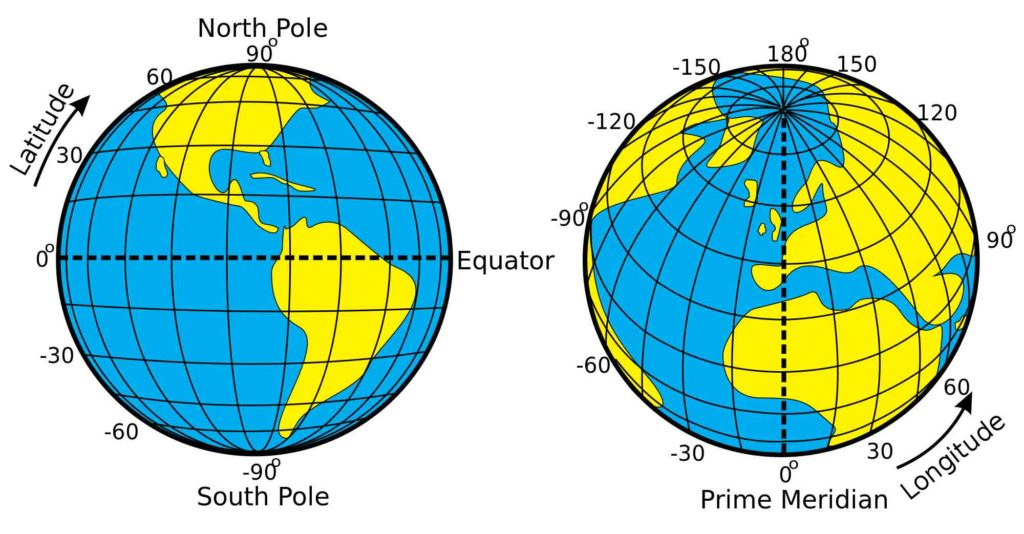“Multiplying the length of the average nautical mile, as indicated by ancient metrology (6,082.56 feet) by 21,600, the number of minutes in a circle, produces the traditional value of the length of the meridian, the great circle passing through the poles.  It proves to be 131,383,296 feet or 24,883.2 miles, the mathematical significance of the mile measure being that 248,832 = 125.”4

It is also interesting to note as John Michell pointed out in The Dimensions of Paradise:

The Roman mile * 25/24 = Greek mile

The Greek mile * 25/24 = English mile

As we see, there are many interconnections.

The Megalithic Yard

“When the late Professor Alexander Thom surveyed over a thousand megalithic structures from Northern Scotland through England, Wales and Western France he was amazed to find that they had all been built using the same unit of measurement.  Thom dubbed this unit a Megalithic Yard (MY = 2.72 feet = 0.829 m) because it was very close in size to an imperial yard, being exactly 2 feet 8.64 inches (82.966 cm).  As an engineer he could appreciate the fine accuracy inherent in the MY but he was mystified as to how such a primitive people could have consistently reproduced such a unit across a zone spanning several hundreds of miles.  The answer that eluded the late Professor lay not in the rocks, but in the stars.  The MY turns out to be much more than an abstract unit such as the modern meter.  It is a highly scientific measure repeatedly constructed by empirical means.  It is based upon observation of three fundamental factors:

1. The orbit of the Earth around the sun
2. The spin of the Earth on its axis
3. The constant mass of the Earth.”5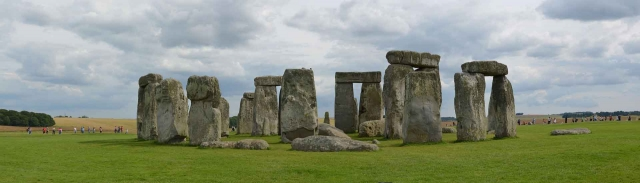“The megalithic stone circles which began to spread throughout the Near East, Northern Europe and Northern Africa are intended to measure the movements of the heavenly bodies.  In the 1950s Professor Thom of Cambridge University first realized that the megalithic stone monuments across the world are constructed according to a common unit of measurement, which he called the ‘megalithic yard’.  This has since been verified by wide-ranging statistical analyses of monuments.  Recently Dr. Robert Lomas of Sheffield University has shown how it was that this unit of measurement was derived to such astonishing unanimity and accuracy in different parts of the world; a pendulum swinging 360 times during the time it takes for a star to move through one of the 360 degrees into which the sky’s dome divides will be exactly 16.32 inches long, which is exactly one half a ‘megalithic yard.’”6

Keith Critchlow, in Time Stands Still, elaborates greatly on Thom’s work.

1 Megalithic Yard = 2.72 feet = 0.82966 meter

1 Egyptian Cubit = 1.72 feet

1 mile = 1,609.344 meters = 1,941 MY

1 nautical mile = 1.15078 mile = 6,076 feet = 1.852 km = 2233 MY

Earth’s diameter = 7,920 miles
Moon’s diameter = 792 megalithic miles
Perimeter of the square containing the circle of the Earth = 31,680 miles
Perimeter of the square containing the circle of the Moon = 3,168 megalithic miles.
Sun’s diameter = 864,000 miles = 316,800 megalithic miles.

“By the time the measurements of Mesopotamia were discovered, by doing various exercises of mathematics on the definitions of the major ancient measurement systems, various people (Jean-Adolphe Decourdemanche in 1909, August Oxe in 1942) came to the conclusion that the relationship between them was well planned.”7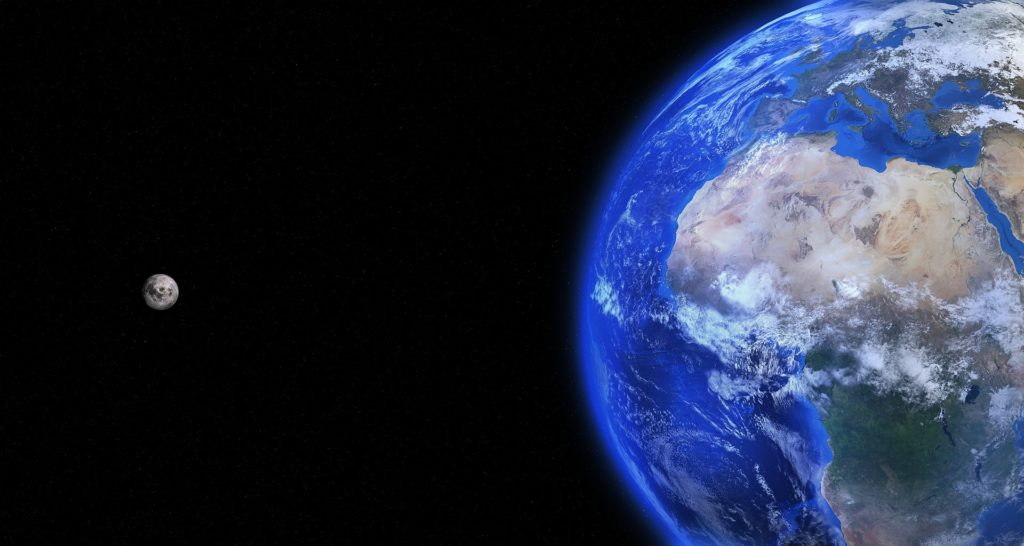The only way these relationships could be well planned was if these ancient people somehow knew the measurements of the Earth from space.

Sacred Metrology Master Diagram – Randall Carlson

Randall Carlson explains, “To measure a thing is to render it sensible and functional, to provide the means by which man engages and interacts with the world.  We must remember, with Protagoras, that ‘Man is the Measure of All Things’ and understand that the system of measure by which we create and build our microcosmic world must of necessity serve as the intermediary between the human scale and the macrocosmic scale to achieve the goal of balance and harmony and effect the integration of the Greater and Lesser.”He then adds, “Measure is the key to revelation.  They key is a universal standard of measure, always presented by a being who is sometimes man, sometimes angel, who is simultaneously a Geometrician, a Builder and, in the original meaning the word ‘angel’ (malak) a Messenger…Through the act of measuring it is seen that each element of the sacred arrangement is linked by a common measure into a system, a whole that is unified through the harmonious integration of the parts.”

There are many ancient and traditional units of measure.

Some of these include:

• Sumerian Shusi (.66 inches)
• Sumerian Foot (20 shusi)
• Sumerian Cubit (30 shusi)
• Egyptian Digit (.729 inches)
• Egyptian Reman (1.2165 feet)
• Royal Cubit (1.72 feet)
• Assyrian Foot (1.08 feet)
• Hebrew Reed (18 inches)
• Palestinian Cubit (25.284 inches)
• Greek foot (12.1375 inches)
• Greek Stade (607 feet)
• Roman Digit (.729 inches)
• Roman Foot (.972 feet)
• Roman Pace (4.86 feet)
• Megalithic Yard (2.72 feet)
• Megalithic Mile (2.727272 statute miles)
• Fathom (6 feet)
• British Pole (16.5 feet)
• Chain (Gunter’s chain) (66 feet)
• Link – 1/100th of a Gunter’s chain
• Furlong (220 yards)
• Statute mile (5820 feet)
• Nautical mile (6080 feet)
• Egyptian Aurora (100 royal cubits on each side)
• Roman Jugerum (165 feet squared or 27,225 sq feet)
• Hindu Nivartana (300 feet on a side)
• Old English Hide (120 acres)
• English acre (1/640 square mile)

Below we will see the Master Diagram of Sacred Metrology as provided by Randall Carlson that incorporates the royal cubit, Palestinian cubit, Roman pace, Megalithic Yard, Modern yard, Assyrian foot and Sumerian foot.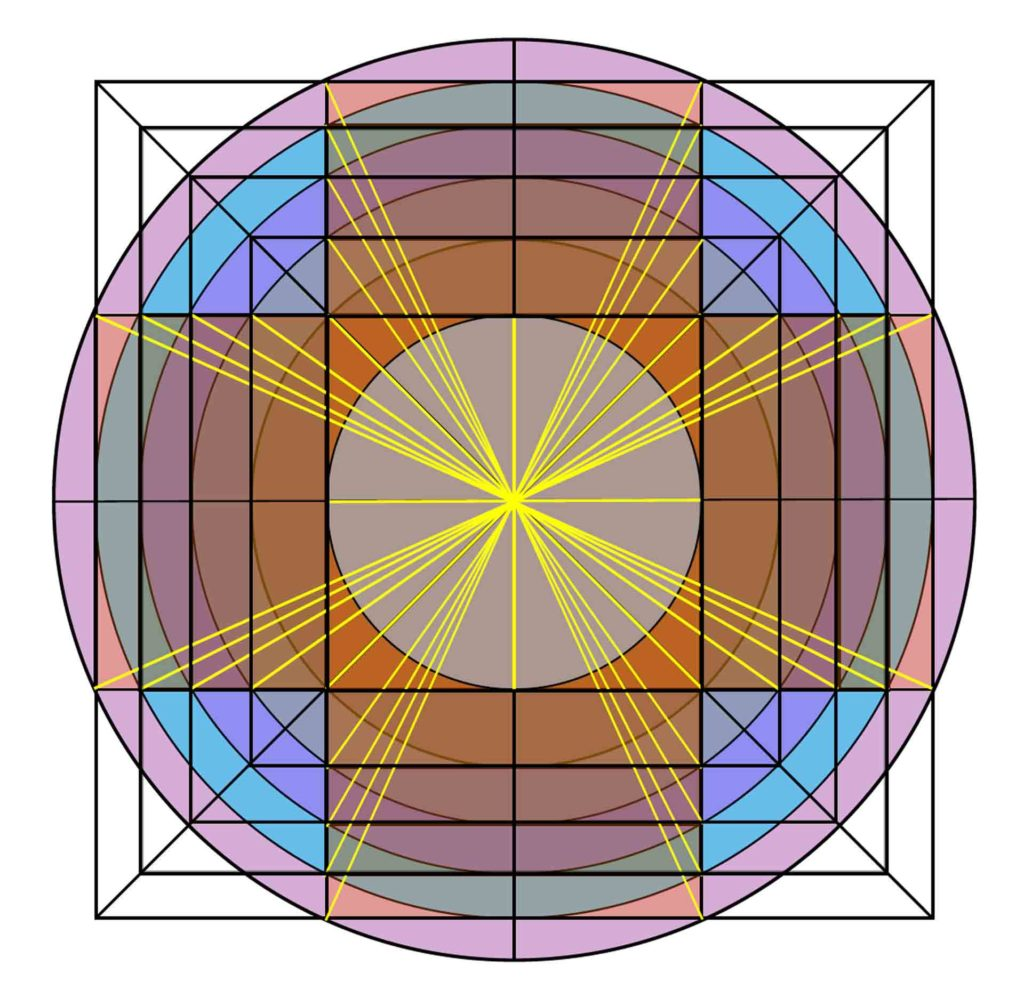The Master Diagram of Sacred Metrology

Circle 1 = The innermost circle

Given that radius of Circle 1 = 1 Egyptian Reman

R of circle 2 = Royal Cubit

R of circle 3 = Palestinian Cubit

R of circle 4 = Roman Pace

R of circle 5 = Megalithic Yard

R of circle 6 = Modern Yard

C of circle 2 = 10 Assyrian ft.

C of circle 3 = 12 Sumerian ft.

R = Radius; C = Circumference

The most significant aspect of ancient metrology is its basis in canonical number.  Canonical number, as we have discussed, relates to the Platonic solids.

The canonical dimensions of the Earth, Sun and Moon are:

• Diameter of sun =      864,000 miles (63 x 4000)
• Radius of sun =          432,000 miles (63 x 2000)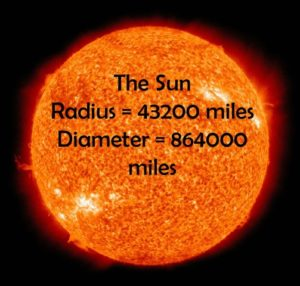• Diameter of moon =      2160 miles (63 x 10)
• Radius of moon =          1080 miles (62 x 30)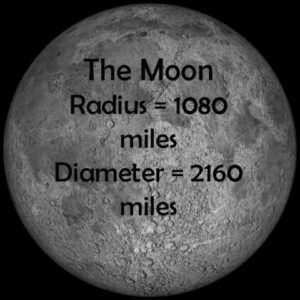• Mean diameter of Earth =   7920 miles (62 x 220)
• Polar diameter =                    99.769 miles
• Equatorial diameter =          365 miles
• Diameter taken through Earth from Tropic of Cancer to Tropic of Capricorn = 7920 miles
• Mean circumference of Earth =          24,883.2 miles (125/10)
• Mean distance from Earth to moon =          237,600 miles (63 x 1100)
• Mean distance from Earth to sun =          93,312,000 miles (66 x 2000)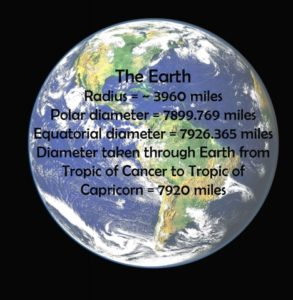• Taking pi = 864/275 = 3.1418
• circumference of moon               =          127 feet
• circumference of earth                =          127 x 11/3 feet
• circumference of sun                   =          127 x 400 feet
• circumference of moon’s orbit    =         127 x 220 feet
• circle made by earth around moon =    127 x 86400 feet

“The conclusion that emerges from these studies is that the ancient philosophers took the key sacred numbers, 12 and 7, put them together in the form of 127, established that number in feet as the measure of the moon’s circumference, and made it the astronomical standard measure of the universe.8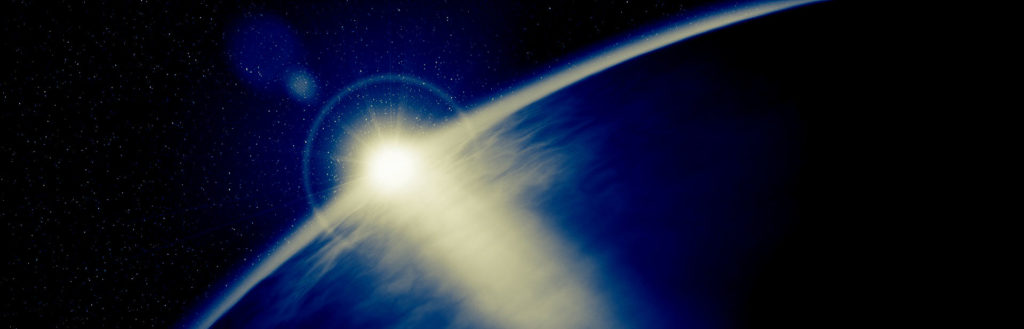Frank Chester, the Chestahedron & Earth Measure

Now that we have reviewed the Platonic solids and seen how their numbers show up in Earth and solar system measure, we will review the 7-sided figure, the Chestahedron, and discuss how it relates to Earth Measure.

We discussed the Chestahedron in Articles 67 (The Heptad) and 197 (The Human Heart).

It is the first known seven-sided solid with faces of equal area.  It was discovered by artist and geometrician Frank Chester.It has: 7 Faces; 7 Vertices; 12 Edges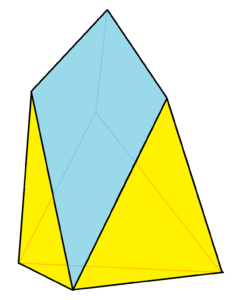Its Faces are 7 in total.  That is, 4 triangles and 3 kite-shaped quadrilaterals.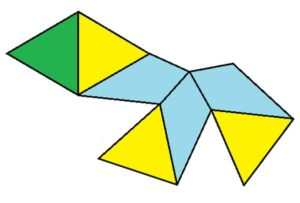Its Vertices are 7 in total.  That is, 4 have 3 edges meeting and 3 have 4 edges meeting.

Its Dual is the dekatria, a 13-sided figure.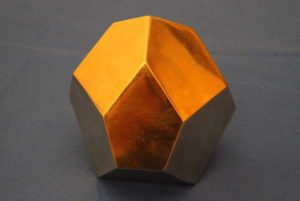The Pentagonal Face of the Dodecahedron:

The two-dimensional, unfolded version relates exactly to a perfect five-pointed star.  The three blue pieces below are three points of a perfect 5-pointed star.Five of the kite shapes, placed with their points together, makes the star pentagon.

This means the Chestahedron can be created by folding up a star pentagon on itself, bringing it into three dimensions.

It also relates to the icosahedron and dodecahedron.

The fundamental uniting factor is the base equilateral triangle.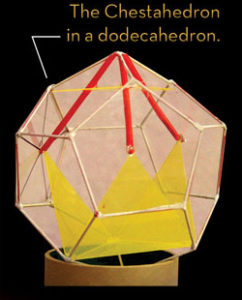Credit: Frank Chester

The Chestahedron can be created in Two Distinct Ways from a Tetrahedron

These are best explained on Frank’s website: http://www.frankchester.com/.

First way: Contractive

Place a tetrahedron inside a cube, and while keeping the boundaries of the cube inviolate, twist the tetrahedron so that one of its points moves along the diagonal of one square face to the opposite corner.

The tetrahedron, at this point, has changed into the octahedron.

There are actually 2 moments, between the transformation of the tetrahedron into the octahedron, that result in the Chestahedron.

The geometry of the Chestahedron is a geometry of motion (vortex motion).

The Chestahedron itself is merely a balanced moment of rest in a whole field of geometric activity that involves all of the Platonic forms.

Second way: Expansive

This begins with the tetrahedron, which is then unfolded like a flower with three petals.

See this in detail at: www.frankchester.com/2009/chestahedron-from-a-tetrahedron/.

The opening of the tetrahedron immediately creates the seven-sided form with the addition of the three kite-shaped faces.

It only remains to unfold the petals to the exact angle at which the area of the kite faces equals the area of the equilateral triangles at angle 94.83°.

This unfolding sequence, traced through time, can be taken further.

If the unfolding of the petals continues past the point at which the Chestahedron arises, a moment comes when the dihedral angle becomes 109.47°.

At this moment it takes the shape of a perfect octahedron with a tetrahedron on top that is exactly the size of the original tetrahedron.

This whole form itself is bounded by a tetrahedron that is exactly twice the size of the original.

The total surface degrees of the Chestahedron (1800°) and its dual, the Decatria (6120°) equals the mean diameter of Earth in statute miles to 99.97% accuracy (NASA 2014).

1800 + 6120 = 7920

The Chestahedron’s Relation to the Earth

Frank Chester found a number of relationships with respect to the size of the inner and outer cores of the Earth including:

• the placement of the auroras
• and the relative size of the core of Earth and moonThe Earth has both an inner and outer core; together they form a sphere 6972 km in diameter.  The Moon has a diameter of 3474 km, which is almost exactly half that of the Earth’s core (~99.7% accuracy).  In other words, two Moons, side by side fit exactly into the Earth’s core.

“Frank Chester found phenomena which appeared to confirm his suggestion that the Chestahedron acts as a fundamental geometric form within our Earth.”9The Chestahedron inside the Earth.  Credit: Frank Chester

The Chestahedron has a cube as its foundation.  In earth dimensions, this cube has the diameter of our Moon.

“In 2008, scientists at Uppsala University in Sweden published findings that appeared to confirm that the core of the earth is a cube (Translator note: Specifically, the round earth’s core has a cubical iron crystalline structure and not a hexagonal one as assumed in older models).

These findings, which are aligned with the idea of a Chestahedron in the earth, offer an explanation why seismic waves travel (through the core) faster along the Earth’s axis (from pole to pole) in comparison to their movement from equator to equator. It can also be shown that notable synchronic lines that join regions of cooler, warmer, and hotter earthly zones in seismic maps correspond essentially to the suggested Chestahedron model within the Earth.”10

Furthermore, “The distribution in the appearance of both the northern lights (aurora borealis) and the southern lights (aurora australis) (on the earth’s surface seen from space) appear to be in alignment with the rings that Frank Chester found in his experiments with the Chestahedron creating water vortices while studying the energetic origin of the human heart.”11

Conclusion

In this article we reviewed the Platonic solids and seen how their numbers show up in the measure of space and time.

In the next article we will discuss the Global Grid, which essentially consists of all five Platonic solids as a nested grid around the Earth.  We will discover the significance of this grid and the implications it has on geology and weather on Earth.

After that we will discuss the Expanding Earth Theory and see how this theory fits into the concept of the global grid.

After that we will move onto the geometry of the solar system, galaxy and universe.

Throughout these articles continue to notice the importance the Platonic solids play.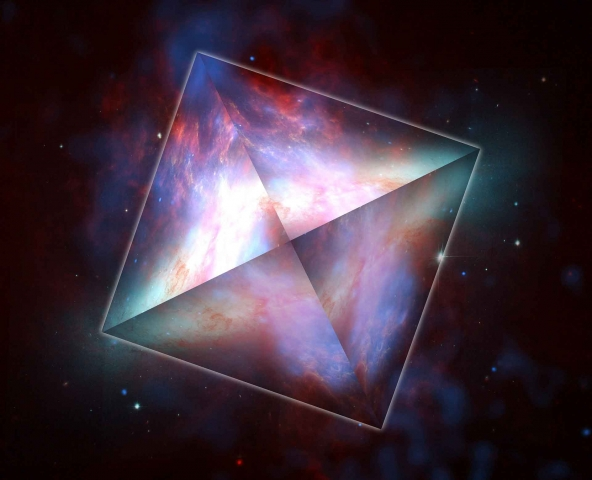1. http://blog.world-mysteries.com/science/numbers-dont-lie-ancient-metrology/
2. ibid.
3. Michell, John, The Dimensions of Paradise, Inner Traditions, 2nd edition, 2008
4. ibid.
5. http://www.robertlomas.com/megyard/index.html
6. Booth, Mark, The Secret History of the World, Overlook Press, 2014
7. http://blog.world-mysteries.com/science/numbers-dont-lie-ancient-metrology/
8. Michell, John, The Dimensions of Paradise, Inner Traditions, 2nd edition, 2008
9. Global Freedom Movement, Chestahedron: The Magic of the Heart Sacred Geometry, https://globalfreedommovement.org/chestahedron-sacred-geometry-revealed/
10. ibid.
11. ibid.